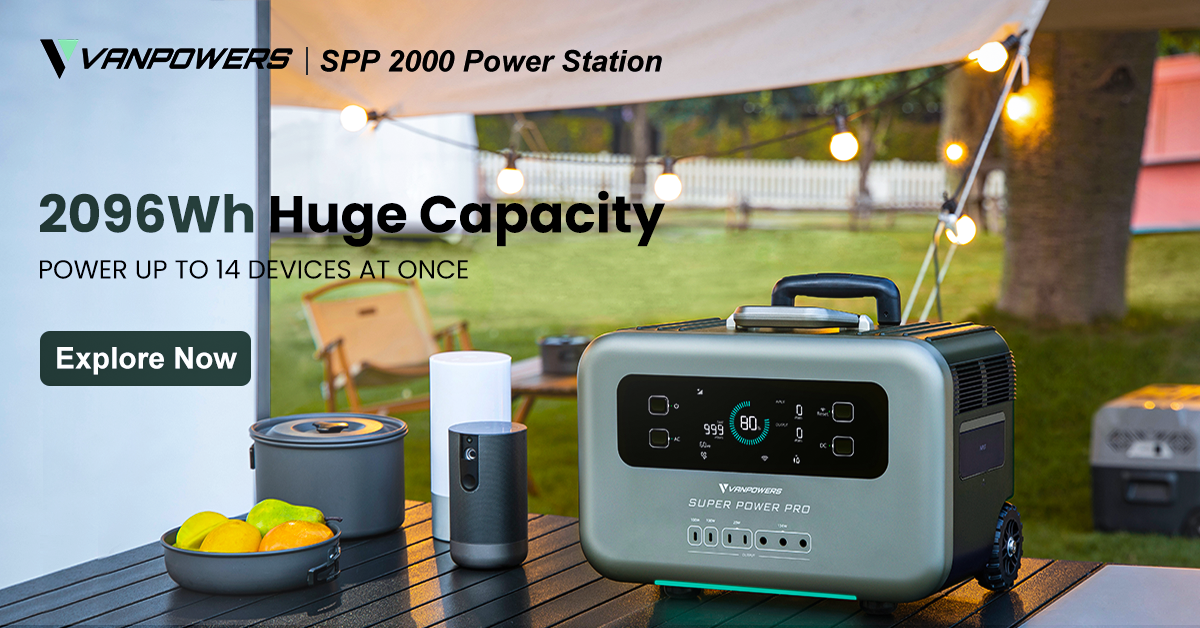## A Guide to Calculating Battery Capacity

Have you ever had a battery go dead unexpectedly? It is crucial to educate oneself on battery capacity if you don't want to find yourself in that situation.

The terms "battery capacity," "amps," "Ah," and "kWh" seem like they’re thrown around everywhere, but they are very important terms to know the meanings of when dealing with batteries or power stations. At the top of the list is battery capacity, which you should learn the fundamentals of before delving into the intricate yet fascinating realm of batteries.

It is feasible to correctly forecast what appliances your battery will be able to power and for how long once you know its size, also called battery capacity. In fact, this information is so crucial that the majority of solar generator producers include the capacity rating in the product name. For instance, the Vanpowers Super Power Pro 2000, one of our products, demonstrates this. This article will cover a variety of topics, including how to determine the ideal battery capacity for your energy requirements.

What is battery capacity?

Appliances are typically rated according to how much power they need to operate (power (W) = voltage x current), but capacity is measured differently. Battery capacity is defined as the total amount of electricity generated by electrochemical reactions in a battery. It is expressed in ampere-hours (Ah), watt-hours (Wh), or kilowatt-hours (kWh). Typically, solar generators are marketed under their energy capacity rating (Wh), while car batteries, or "van life" batteries, are sold under their charge capacity (Ah) classification.

In other words, watt-hours (Wh) indicate energy capacity, while ampere-hours (Ah) indicate charge capacity. Energy capacity (Wh) is used to express the calculated battery capacity. It shows how much energy a battery can store or provide in a single hour. Meanwhile, amperage expresses the amount of electric current flowing to a specific device.

How do you calculate the capacity of a battery?

You may notice that the battery you purchase has its capacity listed in mAh, Ah, Wh, or kWh depending on the type and size of the battery. Small power banks often have their capacity measured in milliampere-hours (mAh), car batteries in ampere-hours (Ah), solar generators in watt-hours (Wh), and residential energy storage systems in kilowatt-hours (kWh). To convert amp-hours to watt-hours, we can use the following equation:

Energy capacity (Wh) = Voltage (V) × Amp-hours (Ah)

Pretend you own a 200Ah 12V battery and would like to know how many Wh it has. You can use the following equation:

200Ah × 12V = 2400Wh

Remember that just because two batteries have the same charge capacity (Ah), that does not necessarily mean they will have the same energy capacity. The voltage may differ, as in the following example:

200Ah 12V battery = 2400Wh

200Ah 24V battery = 4800Wh

How do you calculate the battery’s charge duration after finding its capacity?

Find out how much energy each of your appliances uses. That means you need to find out how many watts your devices need to run. This number is usually listed somewhere on the device itself or its outer packaging.

For example, a fridge and a freezer together might consume 90Wh. If we divide the 2096Wh capacity of the Vanpowers SPP 2000 by 90Wh, we get the following:

2096Wh ÷ 90Wh = 23h

From this result, we see that they would be powered for 23 hours from just one charge.